Related Articles

# Compound Inequalities – Linear Inequalities | Class 11 Maths

• Last Updated : 27 Nov, 2020

A linear inequality is an inequality that includes a linear function.. When two inequalities are joined by the word “and”, the answer occurs when both inequalities are true at an equivalent time. But, when the two inequalities are joined by the word “or”, the solution occurs when either of the inequalities is true. The solution is the combination, or union, of the two individual solutions.

## Compound Inequalities

A compound inequality is an inequality that combines two simple inequalities by either “AND” or “OR“.

The compound inequality with “AND” shows that one of the two statements is true in nature. If there is an overlap of the solution for the individual statements of the compound inequality. Whereas “Or” indicates that, as long as either of the one statement is true, the entire compound sentence is true. It is called the union of the solution sets for the individual statements.

### Compound inequalities: OR

The graph of inequality with an “OR” represents the union as either of the one statement is true, the entire compound sentence is true. The solution of an or inequality, a value has to make only one part of the inequality true. A number is a solution to the compound inequality if the number is a solution to at least one of the inequalities.. The conjunction “or”—when utilized in a compound inequality—forms what’s referred to as a disjunction.

Example: x < 3 OR x > 5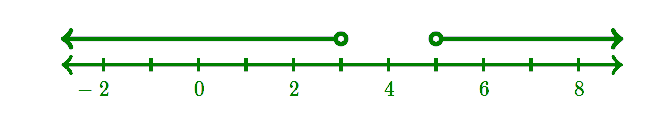The numbers 0 and 6 are both the solutions of the inequality, but the number 4 is not a solution of the given inequality

### Compound inequalities: AND

The graph of a compound inequality with an “and” represents the intersection of the graph of the inequalities.To be an answer of an AND inequality, it must make both parts true. It is the overlap or intersection of the answer sets for the individual statements. A number may be a solution to the compound inequality if the amount may be a solution to both inequalities. A compound inequality that uses the word “and” is understood as conjunction.

Example: x > 0 AND x < 4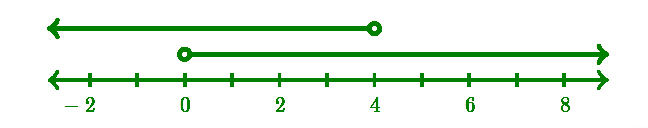So, in this case, 2 is a solution of the compound inequality, but 5 is not because it only satisfies one of the inequalities, not both.

Note: It can also be written as,

0 < x < 4

### Problems on Compound Inequalities

Problem 1: Solve for x in 2x+3≥7  OR  2x+9>11

Solution:

Solving the first inequality for x, we get:

2x+3≥7

2x≥4

x≥2

​ Solving the second inequality for x, we get:

2x+9>11

2x>2

x>1

Graphically, we get: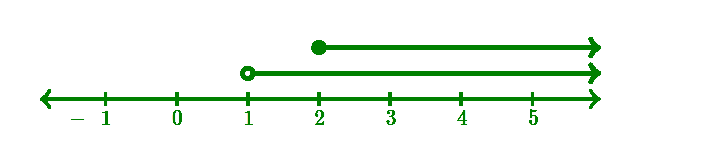The inequality can be expressed as the simple inequality:

x > 1

Problem 2: Solve for y in 2y + 7 < 13 or −3y – 2 <10

Solution:

2y + 7< 13              or              -3y – 2 ≤ 10

+ 7  -7                                      + 2  + 2

2y/2   <  6/2                           -3y/-3   ≥ 12/-3

y < 3                  or                y ≥ -4

The solution is all real numbers.

This number line shows the solution set of y < 3 or y ≥ 4.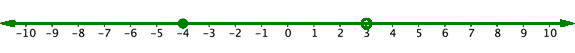Problem 3: Solve  5−3x≤−1 or 8+2x ≤5. Graph the solution and write the solution in interval notation.

Solution:

Given, 5 – 3x ≤ – 1 or 8 + 2x ≤ 5

Solve each inequality.             5 – 3x ≤ – 1                   8 + 2x ≤ 5

−3x ≤ −6                           2x ≤ −3

x ≥ 2  or x ≤ −32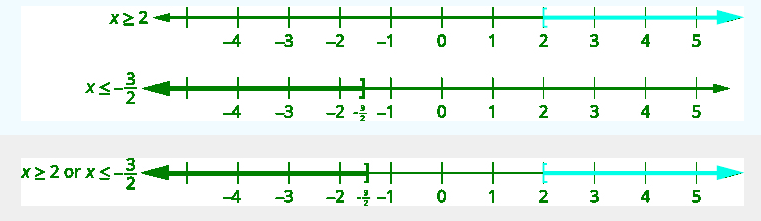Graph numbers that make either inequality true.

The answer for the given inequalities is  (−∞,−32]∪[2,∞)

Problem 4: Solve for x: 3 x + 2 < 14 and 2 x – 5 > -11.

Solution:

To solve this, we will start by solving each equation separately. And as they were joined by  “and,” then it means that, the desired solution is an overlap or intersection.

3x + 2 < 14

Now, Subtract 2 form it and divide it by 3 on both sides

3x + 2 – 2 < 14 – 2

3x/3 < 12/3

x < 4  2x – 5 > -11

Now,

Add 5 to both sides of the equation and divide the equation by 2

2x – 6 + 5 > -11 + 5

2x > -6

x > -3

x < 4 indicates  that all the numbers to the left are 4, and x >( -3 )indicates that all numbers to the right of the inequality are -3. Hence, the solution for this compound inequalities will be, x > –3 and x < 4

Problem 5: Solve 3(2x + 5) ≤ 18 and 2(x – 7) < -6. Graph the solution and write the solution in interval notation.

Solution:

3(2x + 5) ≤18 and 2(x – 7)<−6

Solve each  inequality.  6x+15≤18        2x – 14<-6

6x ≤ 3            2x < 8

x ≤ 12 and x < 4

The answer for the given inequalities is (−∞, 12]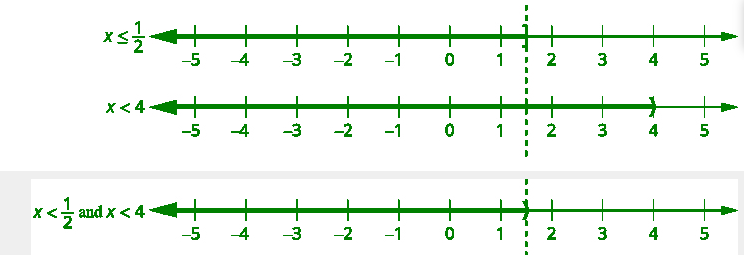Problem 6: Solve the compound inequality. Graph the solution and write the solution in interval notation: 5(3x – 1) ≤ 10 and 4(x + 3) < 8.

Solution:

It is a very simple question and it seems top be solved directly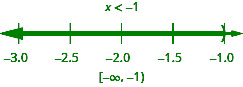Attention reader! Don’t stop learning now. Participate in the Scholorship Test for First-Step-to-DSA Course for Class 9 to 12 students.

My Personal Notes arrow_drop_up